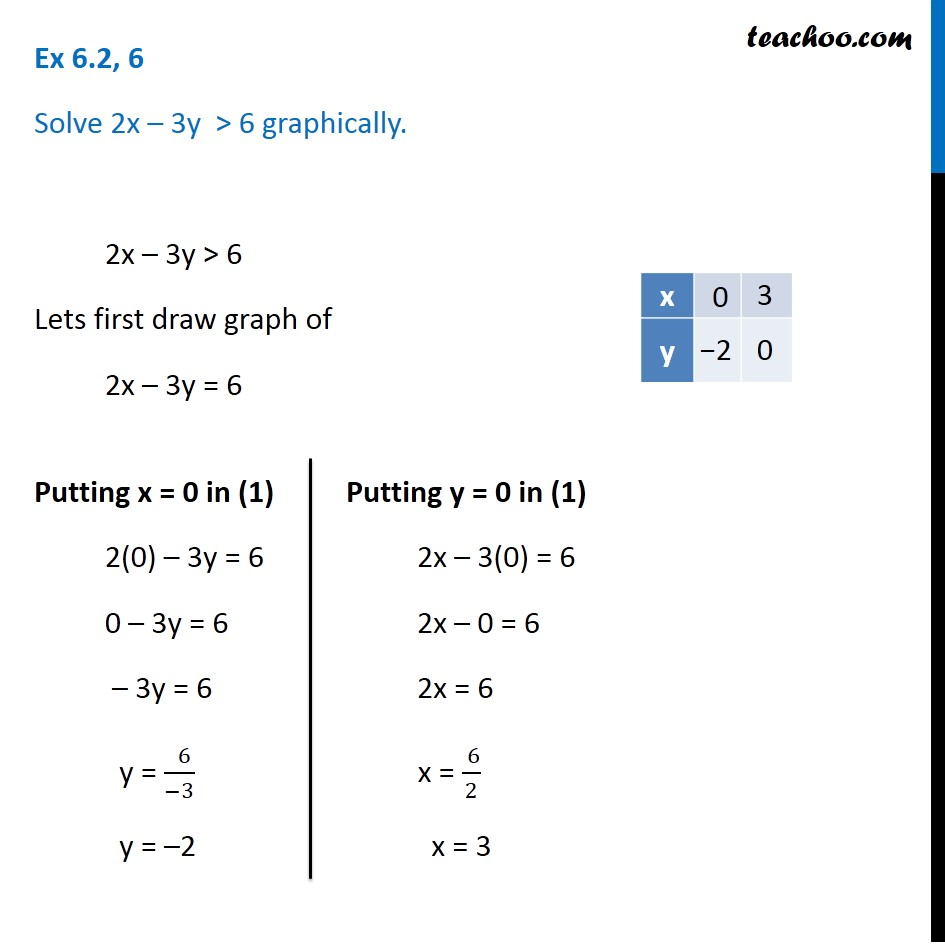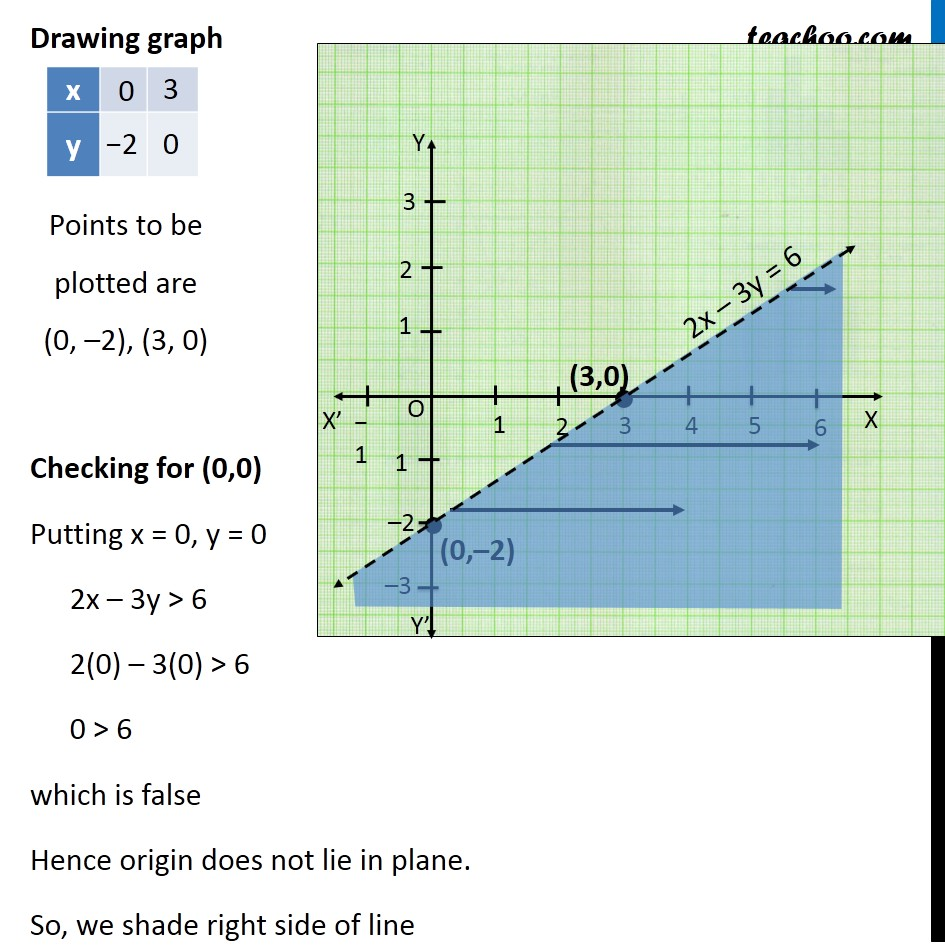Solving Linear Inequality Graphically

Chapter 5 Class 11 Linear Inequalities
Serial order wiseLearn in your speed, with individual attention - Teachoo Maths 1-on-1 Class

### Transcript

Question 6 Solve 2x – 3y > 6 graphically. 2x – 3y > 6 Lets first draw graph of 2x – 3y = 6 VPutting x = 0 in (1) 2(0) – 3y = 6 0 – 3y = 6 – 3y = 6 y = ( 6)/(−3) y = –2 Putting y = 0 in (1) 2x – 3(0) = 6 2x – 0 = 6 2x = 6 x = ( 6)/2 x = 3 Points to be plotted are (0, –2), (3, 0) Drawing graph Checking for (0,0) Putting x = 0, y = 0 2x – 3y > 6 2(0) – 3(0) > 6 0 > 6 which is false Hence origin does not lie in plane. So, we shade right side of line